Maths-
General
Easy

Question

# If α,β then the equation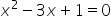with roots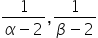will be

##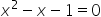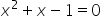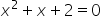none of theseHint:

## The correct answer is:### A quadratic equation, or sometimes just quadratics, is a polynomial equation with a maximum degree of two. It takes the following form:ax² + bx + c = 0where a, b, and c are constant terms and x is the unknown variable.Now we have given the equation as:Here,

Here we used the concept of quadratic equations and solved the problem. We found the sum and product of the roots first and then proceeded for the final answer. Therefore,will be the equation for the roots.#### With Turito Foundation.#### Get an Expert Advice From Turito.